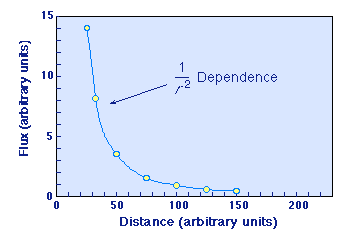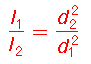Intensity: theInverse Square Law

The intensity of light observed from a source of constant intrinsic luminosity falls off as the square of the distance from the object. This is known as the inverse square law for light intensity.The inverse square law for intensity

Thus, if I double the distance to a light source the observed intensity is decreased to (1/2)2 = 1/4 of its original value. Generally, the ratio of intensities at distances d1 and d2 areHere is a Java virtual experiment illustrating the inverse square law.